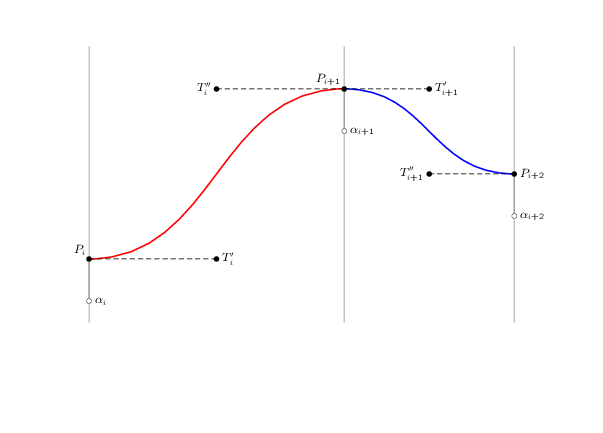# Multiobjective optimization of piecewise spline parameters

 2 I've only just discovered the subject of multiobjective optimization while I'm trying to solve the problem shown/described below. I'd appreciate advise on possible methods of solution (I'm still teaching myself the subject).Given two piecewise continuous cubic curves $$C_1$$ and $$C_2$$ that interpolate three consecutive points $$P_i$$, $$P_{i+1}$$, and $$P_{i+2}$$, I'm looking for the smallest possible values these points, henceforth $$Q_i$$, $$Q_{i+1}$$, and $$Q_{i+2}$$ (each $$Q_j$$ is the new value of $$P_j$$ where $$j = i, i+1, i+2$$ ) such that the gradients of $$C_1$$ and $$C_2$$do not exceed a maximum value $$\Delta_{max}$$. Otherwise stated: Minimize $$P_j - Q_j$$ such that $$\alpha_j \le Q_j \le P_j$$ and the gradient of each curve $$C_j \le \Delta_{max}$$. Note: in reality the problem that I'm trying to solve involves more than two piecewise curves. asked 30 Sep '12, 19:51Olumide 41●1●4 accept rate: 0% I'm struggling to understand the question. Will the C' curves interpolate the Q points rather than the P points? What are the alphas? How do you determine the curves? (An infinite number of cubics interpolate any three points.) (03 Oct '12, 19:19) Paul Rubin ♦♦ The Q points are the new positions of the P points, as such the curves C interpolates the P points pre-optimization as well as the Q points post-optimization. Each curve C is unquely determined by the quartet of T and P points. There is of course an infinite variation of these parameters that satisfy the maximum derivative condition but I'm given to understand that this is the nature of multiobjective optimization. The alphas are lower limits of the points P. (03 Oct '12, 19:36) Olumide
Be the first one to answer this question!
 toggle preview community wiki

By Email:

Markdown Basics

• *italic* or _italic_
• **bold** or __bold__
• image?![alt text](/path/img.jpg "Title")
• numbered list: 1. Foo 2. Bar
• to add a line break simply add two spaces to where you would like the new line to be.
• basic HTML tags are also supported

Tags: